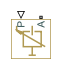# Hydraulic Piston Chamber

(To be removed) Variable volume hydraulic capacity in cylinders

The Hydraulic library will be removed in a future release. Use the Isothermal Liquid library instead. (since R2020a)

## Library

Hydraulic Elements

•## Description

Note

Starting in Release R2014a, you can specify fluid compressibility directly in the hydro-mechanical converter blocks. It is recommended that, instead of using the Hydraulic Piston Chamber block connected to a converter, you use the Compressibility parameter in the converter block dialog box, because the new method provides more accurate results and also because the Hydraulic Piston Chamber block may be removed in a future release.

The Hydraulic Piston Chamber block models fluid compressibility in a chamber created by a piston of a cylinder. The fluid is considered to be a mixture of liquid and a small amount of entrained, nondissolved gas. Use this block together with the Translational Hydro-Mechanical Converter block. The Hydraulic Piston Chamber block takes into account only the flow rate caused by fluid compressibility. The fluid volume consumed to create piston velocity is accounted for in the Translational Hydro-Mechanical Converter block.

The chamber is simulated according to the following equations (see [1, 2]):

`$q=\frac{{V}_{0}+A\left({x}_{0}+x·or\right)}{E}·\frac{dp}{dt}$`
`$E={E}_{l}\frac{1+\alpha {\left(\frac{{p}_{a}}{{p}_{a}+p}\right)}^{1/n}}{1+\alpha \frac{{p}_{a}^{1/n}}{n·{\left({p}_{a}+p\right)}^{\frac{n+1}{n}}}{E}_{l}}$`

where

 q Flow rate due to fluid compressibility V0 Dead volume A Effective piston area x0 Piston initial position x Piston displacement from initial position or Chamber orientation with respect to the globally assigned positive direction. If displacement in positive direction increases the volume of the chamber, or equals 1. If displacement in positive direction decreases the volume of the chamber, or equals –1. E Fluid bulk modulus El Pure liquid bulk modulus p Gauge pressure of fluid in the chamber pα Atmospheric pressure α Relative gas content at atmospheric pressure, α = VG/VL VG Gas volume at atmospheric pressure VL Volume of liquid n Gas-specific heat ratio

The main objective of representing fluid as a mixture of liquid and gas is to introduce an approximate model of cavitation, which takes place in a chamber if pressure drops below fluid vapor saturation level. As it is seen in the graph below, the bulk modulus of a mixture decreases as the gauge pressure approaches zero, thus considerably slowing down further pressure change. At gauge pressures far above zero, a small amount of undissolved gas has practically no effect on the system behavior.Cavitation is an inherently thermodynamic process, requiring consideration of multiple-phase fluids, heat transfers, etc., and as such cannot be accurately simulated with Simscape™ software. But the simplified version implemented in the block is good enough to signal if pressure falls below dangerous level, and to prevent computation failure that normally occurs at negative pressures.

If pressure falls below absolute vacuum (–101325 Pa), the simulation stops and an error message is displayed.

Port A is a hydraulic conserving port associated with the chamber inlet. Port P is a physical signal port that controls piston displacement.

The block positive direction is from port A to the reference point. This means that the flow rate is positive if it flows into the chamber.

### Variables

To set the priority and initial target values for the block variables prior to simulation, use the Initial Targets section in the block dialog box or Property Inspector. For more information, see Set Priority and Initial Target for Block Variables.

Nominal values provide a way to specify the expected magnitude of a variable in a model. Using system scaling based on nominal values increases the simulation robustness. Nominal values can come from different sources, one of which is the Nominal Values section in the block dialog box or Property Inspector. For more information, see Modify Nominal Values for a Block Variable.

## Basic Assumptions and Limitations

• Fluid density remains constant.

• Chamber volume cannot be less than the dead volume.

• Fluid fills the entire chamber volume.

## Parameters

Piston area

Effective piston area. The default value is `5e-4` m^2.

Piston initial position

Initial offset of the piston from the cylinder cap. The default value is 0.

Chamber orientation

Specifies chamber orientation with respect to the globally assigned positive direction. The chamber can be installed in two different ways, depending upon whether the piston motion in the positive direction increases or decreases the volume of the chamber. If piston motion in the positive direction decreases the chamber volume, set the parameter to `Positive displacement decreases volume`. The default value is ```Positive displacement increases volume```.

Volume of fluid in the chamber at zero piston position. The default value is `1e-4` m^3.

Specific heat ratio

Gas-specific heat ratio. The default value is `1.4`.

## Global Parameters

Parameters determined by the type of working fluid:

• Fluid density

• Fluid kinematic viscosity

Use the Hydraulic Fluid (Simscape Fluids) block or the Custom Hydraulic Fluid block to specify the fluid properties.

## Ports

The block has the following ports:

`A`

Hydraulic conserving port associated with the chamber inlet.

`P`

Physical signal port that controls piston displacement.

## References

 Manring, N.D., Hydraulic Control Systems, John Wiley & Sons, New York, 2005

 Meritt, H.E., Hydraulic Control Systems, John Wiley & Sons, New York, 1967

## Version History

Introduced in R2009b

collapse all

### R2023a: To be removed

The Hydraulic library will be removed in a future release. Use the Isothermal Liquid library instead. Blocks in the Isothermal Liquid library provide increased accuracy, usability, and numerical performance.